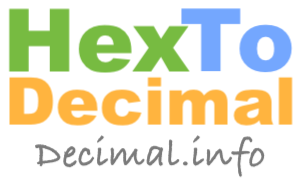0X539 to decimal0X539 is a hexadecimal (hex) number. We can tell it is a hex number because it starts with 0X. Hexadecimal numbers like 0X539 are not often used in daily life, but we see them used for certain things such as html colors, shortening binary numbers, computer error codes, and math exercises.

The hexadecimal number system has 16 symbols (base 16) instead of the decimal system which has 10 numbers (base 10). The hex symbols are 0 1 2 3 4 5 6 7 8 9 A B C D E F where A=10, B=11, C=12. D=13, E=14, and F=15.

Like we said above, the 0X indicates that it is a hex number and that is the only purpose of 0X. Thus, to convert a hex number such as 0X539 to decimal, we only need to look at the symbols after 0X which are 539.

To convert the hex number 0X539 to decimal (or any other hexadecimal number for that matter), you follow these steps:

Step 1) Multiply the last digit by 1, Multiply the second to last digit by 16, Multiply the third to the last digit by 16 x 16, Multiply the fourth to the last digit by 16 x 16 x 16, Multiply the fifth to the last digit by 16 x 16 x 16 x 16 and so on until all the digits are used.

Step 2) Add up all the products you got from Step 1 to get the answer to 0X539 in decimal.

Here is the math using using the steps above showing you how to convert 0X539 to decimal.

9 x 1 = 9
3 x 16 = 48
5 x 16 x 16 = 1280

9 + 48 + 1280 = 1337

That is all there is to it. Here is the answer to 0X539 in decimal:

1337

Hex to Decimal Converter
Here you can convert another hexadecimal number to decimal. Remember hex numbers include numbers 0 through 9 and letters A through F.

0X

0X53A to decimal
Go here for the next hex number on our list that we have converted to decimal.